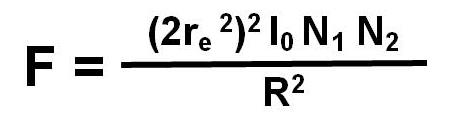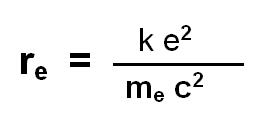Home

# Electromagnetic nature of gravitation.

### The formula does not include masses or charges. It includes only the number N of particles, such as electron or atomic nuclei. She has therefore ascend very good condition to the "world formula". This is a new formula (formula nr. 1) for the four fundamental interactions, the four fundamental forces of physics, in the universe. I could still reasonably easy represent here for individual elements, such as hydrogen or oxygen, in the form of atoms (in low temperatures and without light pressure on Telichen). It is however more complex for diverse elements.Formula no. 1and:The variables are explained below only for gravitation here. F - force of gravitation in [N] (Newton) R - distance between the bodies (in the same meaning as in the present    theories of electromagnetic or gravitational forces) in [m] re - electromagnetic radius of the electron in [m] I0 - density of electromagnetic radiation in [ J / m3 ]     (if the calculations of gravitation between two bodies     affect on the Earth, then "I0" is equal 4,58 10 -6 [N / m2] in distance of 1 AU from the Sun measured (Solar constant (1360,8 +- 0,5 [W m-2]/c - speed of Light)) N1 - the total number of light scattering particles in the first body N2 - the total number of light-scattering particles in the second body k - Coulomb constant equal 9 109 [V * m / A * s] e - elementary charge (charge of the electron) equal 1,9 10-19[C] me - mass of the electron equal 9,1 10-31 [kg] c - speed of light in [m / s] ε0 - electric field constant equal 8,89 10-12 [F / m]

Here "light" in importance of electromagnetic waves

My electromagnetic theory of gravitation is easy to extend to a unified theory of all forces in the universe. I have referred to as "electromagnetic theory of the swing force" because the value of - 2 x (electromagnetic radius of electron) times two(2*re2)- a scattering cross-section for inelastic scattering of electromagnetic waves is on a elemental particle or nucleus. The size "I0" represents the density of electromagnetic waves All this leaves no doubt that gravitation is a result of the interaction of electromagnetic waves and matter. I have given "Constants" the values of some physical food assistants that I have used here for calculations.